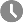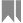# My jQuery SnippetsPublished July 19, 2014Coding > JavaScriptThe following is a collection of my jQuery snippets. These snippets are little things I don't want to forget or helpful scripts I've come across during work. This article isn't meant to be a tutorial, but I placed helpful links and JSFiddle's for further assistance.

## Basics

jQuery starting template

``````<script src="http://code.jquery.com/jquery-latest.min.js" type="text/javascript"></script>
<script>
// insert code here
});
</script>``````

Creating a function

JSFiddle
``````function sample(x, y) {
\$('<p>This is a ' + x + ' test. ' + y + '</p>').prependTo('p');
}
sample('simple', 'It\'s easy.');``````

If statement

``````if (divWidth == '1') {
// do something
} else {
// do something else
}``````

If element contains something

``````if (\$(this).find('ul').length > 0) {
// do something
}``````

Top

## CSS

Single CSS style

``\$('.demo td:nth-child(3)').css('width','80px');``

Multiple styles

``\$('.demo tr td:nth-child(2)').css({'width':'580px','padding-top':'15px'});``

Multiple styles packaged into a variable

``````var styles = {'margin-top':'100px', 'font-weight':'bold', 'font-size':'30px'};
\$('.demo').css(styles);``````

Top

## Loop

The following is the loop script. In the sample, “10” would be the amount of times the loop repeats. Keep in mind, though, that if you just want to run jQuery through a series of like elements then it's better to use the Each function.

``````for(var i = 0; i < 10; i++) {
// do something
}``````

The loop amount could be set as a variable as well.

``````var loopamount = 10;
for(var i = 0; i < loopamount; i++) {
// do something
}``````

Top

## Data Attributes

Usage in HTML

``<a href="demo.html" data-country="united states" data-state="florida">Sample</a>``

Setting it to a variable

``var sample = \$('#dropdown').data('country')``

Changing it

``\$('a').attr('data-country', 'mexico');``

In an If statement, if data equals x

``````if (\$(this).data('door') == 'open') {
// do something
}``````

In an If statement, if data is not equal to x

``````if (\$(this).data('door') != 'open') {
// do something
}``````

Target all elements with specific data attribute

``````\$('[data-demo]').on('click', function() {
// do something
});``````

Target all elements with specific data attribute & its text

``````\$('[data-demo="some-text"]').on('click', function() {
// do something
});``````

Top

## Avoiding parent().parent().parent()...

Avoid typing parent() over and over with “parents()” and “eq()”. Type parents() then put eq() with the number of parent()'s -1. For example, if you're using 3 parent()'s do the following.

``\$(this).parents().eq(2).find('p');``

Top

## Using Index and Eq

This is important to know for targeting elements. To pass around and use an element's index, you need to use CSS's eq.

JSFiddle
``````var demo = \$(this).index();
\$('li:eq(' + demo + ')').toggleClass('red');``````

Top

This is actually pure JavaScript, not jQuery. This script makes a side navigation stick to the top of the page upon scroll. This is appropriate for when you have a column reserved entirely for the navigation to pass through. Sticky navigations are a popular effect - there are many sticky plugins available if this script doesn't work out for you.

JSFiddle
``````var menu = document.querySelector('.menu')
} else {
}
});``````

Forking from above, if the navigation that you need to sticky is between the header and content then that requires a few extra steps. The following script creates an empty DIV to correct an awkward jump that occurs when the navigation switches to its sticky state.

JSFiddle
``````var menu = document.querySelector('.menu');
var placeholder = document.createElement('div');
}
});``````

Top

This script toggles an animation that fades and expands/collapses at the same time.

JSFiddle
``````\$.fn.slideFadeToggle  = function(speed, easing, callback) {
return this.animate({opacity: 'toggle', height: 'toggle'}, speed, easing, callback);
};
\$("#something").click(function() {
});``````

However, you can't use the above script when you're using a hover because you'll run into cue problems. In that case, use HoverFlow and the following script.

JSFiddle
``````\$('.button').hover(function(e) {
\$(this).next().hoverFlow(e.type, { opacity: 'toggle', height: 'toggle' }, 'fast');
}, function(e) {
\$(this).next().hoverFlow(e.type, { opacity: 'toggle', height: 'toggle' }, 'fast');
});``````

Top

## Hover or Click Button

This is useful for responsive sites.

JSFiddle
``````var hoverOrClick = function () {
// reveal something
}
\$('.options').click(hoverOrClick).hover(hoverOrClick);``````

However, the above code doesn't play nice with multiple animations such as with my Fade+Expand Animation. Using HoverFlow, the following script would make a hover/click button work with multiple animations.

JSFiddle
``````// Hover
return this.hoverFlow(e.type, {opacity: 'toggle', height: 'toggle'}, 'fast', 'linear', function() {});
};
var hoverExpand = function (e) {
}
\$('.dropdown').hover(hoverExpand);
// Click
\$('div').click(function() {
if (\$(this).find('ul').is(':visible')) {
\$(this).find('ul').hide();
} else {
\$(this).find('ul').show();
}
});``````

Top

## Current Page Style & :not

Using :not can be very useful with jQuery. One such use is when you're using a current-page style in a jQuery animated navigation. You can apply the jQuery animation on every navigation link except the one you set as the current page. Also, in this sample I'm using the Color animation jQuery-plugin for an elegant background color transition.

JSFiddle
``````\$('#navigation li:not(#current) a').hover(
function () {
\$(this).animate({
'background-color': '#00bff3',
}, 'fast', 'linear', function () {});
},
function () {
\$(this).animate({
'background-color': '#0072bc',
}, 'fast', 'linear', function () {});
});``````

Top

## Scroll Page

Scroll to bottom of page

JSFiddle
``````\$('html, body').animate({scrollTop:\$(document).height()});
return false;``````

Scroll to top of page

JSFiddle
``````\$('html, body').animate({scrollTop:0});
return false;``````

Scroll a little bit

``````\$('html, body').animate({scrollTop:'-20px'}, 'slow');
return false;``````

Scroll to an element

``````\$('html,body').animate({ scrollTop: \$('#target').offset().top}, 'medium');
return false;``````

In addition to above, in some cases you may need to scroll higher to the parent element.

``````\$('html,body').animate({ scrollTop: \$(this).parent().parent().offset().top}, 'medium');
return false;``````

Top

## Appear in Domino Effect

A quick script to make a group of elements fade in one by one with a domino effect animation.

JSFiddle
``````\$('div').hide();
\$('div').each(function(i) {
});``````

Top

## Basic Timeline Animation

This is the most basic method of timeline based animation. The number you enter for the delay would be the element's position in the timeline. However I don't recommend this script for complex animations. There are several great jQuery animation plugins out there such as Spritely and Collie.

JSFiddle
``````block1 = \$('#block1');
block2 = \$('#block2');
block1.delay(400).animate({'left':300}, 'slow');
block2.delay(400).animate({'top':200}, 'slow');
block2.delay(700).animate({'width':200,'height':200}, 'slow');
block1.delay(900).animate({'left':-200,'opacity':0}, 'slow');
block2.delay(1000).animate({'top':-200,'height':-200,'opacity':0}, 'slow');``````

Top

## Random Element

Grab a random element from a group.

JSFiddle
``````var count = \$('.blocks div').length;
var randomer = Math.ceil(Math.random() * count)
\$('.blocks div').each(function () {
var pos = \$(this).index() + 1;
\$(this).hide();
if (pos == randomer) {
\$(this).show();
}
});``````

Top

## Unclickable Children

When you've applied a click event to an element, this script disables the click event for child elements. For example, this is useful when you have an accordion with buttons inside the click area.

JSFiddle
``````\$('h2').click(function () {
\$(this).toggleClass('demo');
});
\$('h2 span').click(function (e) {
e.stopPropagation();
});``````

Top

## Hide Element If User Clicks Outside

Hide an element if the user clicks anywhere on the page outside of it. This script is useful if you're creating a DIV driven form.

JSFiddle
``````\$(document).mouseup(function (e) {
var container = \$("div");
if (!container.is(e.target) && container.has(e.target).length === 0) {
container.hide();
}
});``````

Top

I usually prefer making a DIV or other element a button for jQuery events, but sometimes I use an anchor. In the JSFiddle I have you scroll down to the bottom on the page to show you that the page doesn't refresh when you click the anchor.

JSFiddle
``````\$('a').on('click', function(e) {
e.preventDefault();
})``````

Top

When working with jQuery UI, jQuery Mobile, AJAX, or other content dynamically slapped onto the page after it has loaded, a common problem is being unable to add click events to such content. This is resolved with the following; it's called binding.

``````\$(document).on("click", ".sample-div", function(e) {
// enter action here
});``````

Top

## Rounding Numbers

Rounding up, rounding down, and rounding to the nearest 10.

JSFiddle
``````\$("#roundDown").text('Rounded down: ' + Math.floor(number / 10) * 10);
\$("#roundUp").text('Rounded up: ' + Math.ceil(number / 10) * 10);
\$("#roundNearest").text('Rounded to nearest: ' + Math.round(number / 10) * 10);``````

Top

## Conditional Formatting in Tables

Color a table cell if a number is greater than or less than the number you set.

JSFiddle
``````\$('td').each(function () {
if(\$(this).text() >= 200) {
\$(this).css({'color':'red','font-weight':'bold'});
}
if(\$(this).text() <= 100) {
\$(this).css({'color':'blue','font-weight':'bold'});
}
});``````

Color a table cell based on the result of comparing two cells.

JSFiddle
``````\$(".total").each(function() {
var year1 = parseInt(\$(this).prev().prev().text().replace(/[^0-9\.]+/g,""), 10);
var year2 = parseInt(\$(this).prev().text().replace(/[^0-9\.]+/g,""), 10);
if (year1 > year2) {
\$(this).css({'color': 'red'});
} else {
\$(this).css({'color': 'green'});
}
});``````

Top

Removing currency formatting and then adding it back. To add the currency formatting I'm using the Format Currency Plugin.

JSFiddle
``````var source = \$('#source').text();
var removeCurrency = source.replace(/[^0-9\.]+/g,""); // Removes currency
\$('#removed').text(removeCurrency);
\$('#removed').formatCurrency('#return'); // Adds currency and sends back to DOM``````

Top

## Collect Row as Array

Grabs all the items in a table row and compiles it into an array.

JSFiddle
``````var pets = [];
\$('#secondRow td').each(function(i, elem) {
pets.push(\$(elem).text());
});
\$('p').html('Your array contains: ' + pets);``````

Top

## Sum of Every Table Row

Runs through every table row and adds up the total.

JSFiddle
``````\$('tbody tr').each(function() {
row_total = 0;
\$(this).find('td:not(.total)').each(function() {
row_total += parseInt(\$(this).text(), 10);
});
\$('.total',this).text(row_total);
});``````

Top

## Reveal Content Based on Radio Selection

The basis of making a form conditional.

JSFiddle
``````\$("input[name\$='search']").click(function(){
\$(".location").slideDown("medium");
}
\$(".location").slideUp("medium");
}
});
\$(".location").hide();``````

Top

## Checkbox Manipulation

Do something if a checkbox is checked.

JSFiddle
``````\$('input[type="checkbox"]').click(function() {
if (\$(this).is(':checked')) {
// do something
}
});``````

Check or uncheck a checkbox.

``````\$('.myCheckbox').prop('checked', true); // Check it
\$('.myCheckbox').prop('checked', false); // Uncheck it``````

Top

## Expanding Textarea to Fit Text

Expands the height of the textarea to fit the text as the user is typing.

JSFiddle
``````\$("#demo").mousemove(function (e) {
var myPos = \$(this).offset();
myPos.bottom = \$(this).offset().top + \$(this).outerHeight();
myPos.right = \$(this).offset().left + \$(this).outerWidth();
if (myPos.bottom > e.pageY && e.pageY > myPos.bottom - 16 && myPos.right > e.pageX && e.pageX > myPos.right - 16) {
\$(this).css({ cursor: "nw-resize" });
} else {
\$(this).css({ cursor: "" });
}
})
.keyup(function (e) {
while (\$(this).outerHeight() < this.scrollHeight + parseFloat(\$(this).css("borderTopWidth")) + parseFloat(\$(this).css("borderBottomWidth"))) {
\$(this).height(\$(this).height() + 1);
};
});``````

Top

## Simple Spinner

A spinner uses arrow buttons to increment a number. In this spinner, the left or right arrow disables if the minimum or maximum number is the current number. Spinners are good for mobile sites.

JSFiddle
``````// Set variables
var currentValue = parseInt(\$('input').val(), 10);
var minNum = 1;
var maxNum = 4;

// On page load, disable arrow if min or max is default value
if (currentValue == minNum) {
}
if (currentValue == maxNum) {
}

// Click events
\$('.leftArrow').click(function() {
if (currentValue != minNum) {
currentValue--;
\$('input').val(currentValue);
\$('.rightArrow').removeClass('dim');
}
if (currentValue == minNum) {
}
});
\$('.rightArrow').click(function() {
if (currentValue != maxNum) {
currentValue++;
\$('input').val(currentValue);
\$('.leftArrow').removeClass('dim');
}
if (currentValue == maxNum) {
}
});``````

Top

## Dynamically Sized Columns

This script resolves the need to have 100% height on an element (or row of elements), but the height takes into account elements above and/or below that have a fixed height. This is responsive because the faux 100% height is recalculated every time the user adjusts the browser window. In the JSFiddle resize the browser to see it in action.

JSFiddle
``````var callback = function () {
var windowSize = \$(window).height();
var wrapperHeight = windowSize - headingHeight;
\$('.wrapper div').height(wrapperHeight);
};
\$(window).resize(callback);``````

Top